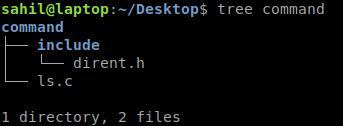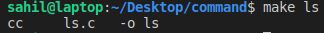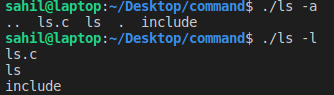×

Search anything:

# Implementation of ls command in C

#### Software Engineering C ProgrammingGet this book -> Problems on Array: For Interviews and Competitive Programming

Today we are going to discuss an important command of the bash shell, which is "ls" command. This command is used for listing the files and directories present in an user space.

We are going to implement that using the "dirent" header file.

## Initial Setup

Make a directory named "command" and inside that make a directory named "include", also in the include/ directory place the header file "dirent.h".
Also make a file "ls.c" where our main implementation code will reside.After the initial setup, we can proceed to the implementation part of the program.

### Implementation

To begin with the work, we will first have to include the header files in the "ls.c" file,

We would need 4 header files, namely:

• stdio.h
• dirent.h
• errno.h
• stdlib.h
``````//Used for basic input/output stream
#include <stdio.h>
//Used for handling directory files
#include <dirent.h>
//For EXIT codes and error handling
#include <errno.h>
#include <stdlib.h>
``````

After including the files, we will implement the core logic of the command, for this we will create a function named `_ls(const char *dir, int op_a, int op_l)`, so let's see the implementation of the function,

First we will declare the function as,

``````void _ls(const char *dir,int op_a,int op_l)
{
//Function logic here
}
``````

Then we will write the function body, so for the first step we will create a "dirent" object for using its functions, and also the DIR object for file navigation.

``````	struct dirent *d;
DIR *dh = opendir(dir);
``````

Then if the file is not present in the directory or the pointer is invalid we will throw an error and exit from the program.

``````if (!dh)
{
if (errno = ENOENT)
{
perror("Directory doesn't exist");
}
else
{
//If the directory is not readable then throw error and exit
}
exit(EXIT_FAILURE);
}
``````

Else, while the directory is readable we will print until theres no file or directory left in the folder.

``````	//While the next entry is not readable we will print directory files
while ((d = readdir(dh)) != NULL)
{
//If hidden files are found we continue
if (!op_a && d->d_name == '.')
continue;
printf("%s  ", d->d_name);
if(op_l) printf("\n");
}
``````

Here the variable `op_a` specifies that whether we want to list all files in the directory(which are hidden) or only the unhidden files, so we can pass an argument while executing the command "-a".

The variable `op_l` specifies that whether we want to list all files in the normal form without going to next line and by keeping the hidden files hidden or not.

Also the above code prints the `d->d_name` which is the file name or the directory name followed by a `\n` sign.

Now we will write the `main` function code,

``````int main(int argc, const char *argv[])
{
//Main Code here
}

``````

Inside the main function code we will take arguments and code accordingly to the options provided,

If no arguments are provided then we will just use the default `ls` command on the directory,

``````	if (argc == 1)
{
_ls(".",0,0);
}
``````

Else with options we will follow another approach wherein we would take decision according to `-a` option or `-l` option.

``````else if (argc == 2)
{
if (argv == '-')
{
//Checking if option is passed
//Options supporting: a, l
int op_a = 0, op_l = 0;
char *p = (char*)(argv + 1);
while(*p){
if(*p == 'a') op_a = 1;
else if(*p == 'l') op_l = 1;
else{
perror("Option not available");
exit(EXIT_FAILURE);
}
p++;
}
_ls(".",op_a,op_l);
}
}

``````

So the implementation of the `ls` command is complete.

### Final Code:

The `ls.c` file is:

``````//Used for basic input/output stream
#include <stdio.h>
//Used for handling directory files
#include <dirent.h>
//For EXIT codes and error handling
#include <errno.h>
#include <stdlib.h>

void _ls(const char *dir,int op_a,int op_l)
{
//Here we will list the directory
struct dirent *d;
DIR *dh = opendir(dir);
if (!dh)
{
if (errno = ENOENT)
{
perror("Directory doesn't exist");
}
else
{
//If the directory is not readable then throw error and exit
}
exit(EXIT_FAILURE);
}
//While the next entry is not readable we will print directory files
while ((d = readdir(dh)) != NULL)
{
//If hidden files are found we continue
if (!op_a && d->d_name == '.')
continue;
printf("%s  ", d->d_name);
if(op_l) printf("\n");
}
if(!op_l)
printf("\n");
}
int main(int argc, const char *argv[])
{
if (argc == 1)
{
_ls(".",0,0);
}
else if (argc == 2)
{
if (argv == '-')
{
//Checking if option is passed
//Options supporting: a, l
int op_a = 0, op_l = 0;
char *p = (char*)(argv + 1);
while(*p){
if(*p == 'a') op_a = 1;
else if(*p == 'l') op_l = 1;
else{
perror("Option not available");
exit(EXIT_FAILURE);
}
p++;
}
_ls(".",op_a,op_l);
}
}
return 0;
}
``````

Now, to use the command we will first compile the `ls`, for this we use the `make` command.Link to the direct library is : github.com/tronkko/dirent

Hence, the command works and this completes our implementation of ls command of Linux in C. Enjoy.#### Sahil Silare

B.Tech Student in Computer Science at National Institute of Technology (NIT), Raipur (2019 - 2023) | Intern at OpenGenus

Implementation of ls command in C